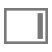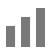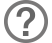# Specify Calculation dialog box

This dialog box appears so that you can build a formula for a calculation or calculated field.

1. If necessary, for Evaluate this calculation from the context of, choose a table from the list.

Setting the context for a calculation is only necessary when you're creating a calculation field in a source table that has two or more occurrences in the relationships graph. The choice you make may affect the calculation results, particularly if your calculation will include fields in related tables.

For more information on the use of context in calculations, see Choosing the evaluation context for a calculation field.

2. Build a formula for your calculation using field references, operators, constant values, and functions.

In the calculation pane, click where you want the item to appear, then:

3.  To add Do this A reference to a field Do one of the following: Begin typing the field’s table, choose the table from the list, begin typing the field name, then choose the field name from the list the appears. In the fields pane, double-click the field name. To display field names from a different table, choose the table from the tables list. An operator or an exponent Do one of the following: Type an operator. Click an operator in the list to the right of the calculation pane. A constant value Type the value. A function Do one of the following: Begin typing the function name, then choose a function name from the list that appears. In the functions pane, double-click the function. In the calculation pane, replace the placeholder parameter with a value or expression.
4. Specify calculation options for the field.
5.  To Do this Set the field type of the result Choose a data type for Calculation result is . Choose the correct type for the result you want. See About choosing a field type. Make a calculated field repeating For Number of repetitions, type the number of repetitions. See Defining repeating fields. Prevent calculation if all referenced fields are empty Select Do not evaluate if all referenced fields are empty. When enabled, FileMaker Pro does not evaluate a calculation if all fields used by the calculation are empty. This typically improves performance.

Important  To avoid confusion when using dates in FileMaker Pro, always define calculation formulas to enter four-digit years. See Entering dates with two-digit years.

6. To select indexing and storage options for the field, click Storage Options, then choose options in the Storage Options dialog box.

## Notes

• To show or hide the functions pane, click.
• To filter the list of fields or functions in the fields pane or the functions pane, type the field name or function name in the search box.
• To change the way fields or functions are displayed in the fields pane or the functions pane, click.
• To view information about a function, click the function in the functions pane. A description appears below the list. To view more information, click.